NEET  >  Stoichiometry & Stoichiometric Calculations and Concentration Terms

# Stoichiometry & Stoichiometric Calculations and Concentration Terms | Chemistry Class 11 - NEET

 1 Crore+ students have signed up on EduRev. Have you?

Stoichiometry

Stoichiometry is the calculation of the quantities of reactants and products involved in a chemical reaction.
Following methods can be used for solving problems:
(a) Mole Method (For Balance reaction)
(b) POAC method (Balancing not required but common sense, use it with slight care)
(c) Equivalent Concept

➢ Concept of Limiting Reagent

Limiting Reagent: It is a very important concept in chemical calculation. It refers to reactant which is present in minimum stoichiometry quantity for a chemical reaction.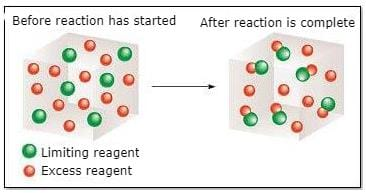Concept of Limiting Reagent

It is the reactant consumed fully in a chemical reaction. So all calculations related to various products or in the sequence of reactions are made on the basis of limiting reagent.
It comes into the picture when a reaction involves two or more reactants. For solving any such reaction, the first step is to calculate L.R.

Calculation of Limiting Reagent:
(a) By calculating the required amount by the equation and comparing it with the given amount. [Useful when only two reactants are there].

(b) By calculating the amount of any one product taking each reactant one by one irrespective of other reactants. The one giving the least product is limiting reagent.

(c) Divide given moles of each reactant by their stoichiometric coefficient, the one with the least ratio is the limiting reagent. [Useful when the number of reactants are more than two or two.

➢ Percentage Yield

The percentage yield of product is: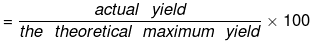The actual amount of any limiting reagent consumed in such incomplete reactions is given by:
% yield × given moles of limiting reagent [For reversible reactions].

Example 1. A compound which contains one atom of X and two atoms of Y for each three atoms of Z is made by mixing 5 gm of X, 1.15 × 1023 atoms of Y and 0.03 mole of Z atoms. Given that only 4.40 gm of compound results. Calculate the atomic weight of Y if atomic weight of X and Z are 60 and 80 respectively.
Solution. Moles of x = 5/60 = 1/12 = 0.083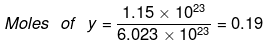Moles of z = 0.03
x + 2y + 3z → xy2z3
For limiting reagent, 0.083/1 = 0.083
0.19/2 = 0.095 , 0.03/3 = 0.01
Hence z is limiting reagent
wt of xy2z3 = 4.4 gm = moles × molecular wt.
Moles of xy2z3 = 1/3 × 0.03 = 0.01
300 + 2m = 440
⇒ 2m = 440 - 300 ⇒ m =70

➢ POAC Rule

POAC is the simple mass conservation:
KClO3 → KClO2
Apply the POAC on K.
moles of K in KClO3 = moles of K in KCl
1 × moles of KClO3 = 1 × moles of KCl
moles of KClO3 = moles of KCl
Apply POAC on O
moles O in KClO= moles of O in O2
3 × moles of KClO3 = 2 × moles of O2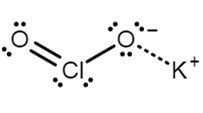Structure of KCLO3

Example 2. In the gravimetric determination of phosphorous, an aqueous solution of dihydrogen phosphate ion (H2PO4-) is treated with a mix of ammonium & magnesium ions to precipitate magnesium ammonium phosphate MgNH4 PO4.6H2O. This is heated and decomposed to magnesium Pyrophosphate, Mg2P2O7 which is weighted. A solution of H2PO4- yielded 1.054 gm of Mg2P2O7 what weight of NaH2PO4 was present originally.
NaH2PO4 → Mg2P2Oapply POAC on P
Solution. Let wt of NaH2PO4 = w gm
moles of P in NaH2PO4 = moles of P in Mg2P2O7
w/120 × 1 = 1.054/232 × 2
w = 1.054 x 120/232 × 2 = 1.09 gm

Dulong's and Petit's Law
Atomic weight × specific heat (cal/gm°C) ∝ ≌ 6.4
Gives approximate atomic weight and is applicable for metals only.
Take care of units of specific heat.

Example 3. 7.5 mL of a hydrocarbon gas was exploded with an excess of oxygen. On cooling, it was found to have undergone a contraction of 15 mL. If the vapour density of the hydrocarbon is 14, determine its molecular formula. (C = 12, H = 1)

Solution.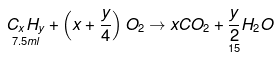on cooling the volume contraction = 15 ml
i.e The volume of H2O (g) = 15 ml
V.D of hydrocarbon = 14
Molecular wt. of CxHx = 28
12x + y = 28 ...(1)
From reaction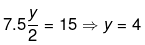12 x + 4 = 28
12 x = 24
x = 2
Hence Hydrocarbon is C2H4.

Example 4. Calculate the weight of FeO produced from 2g VO and 5.75 g of Fe2O3. Also report the limiting reagent.
VO + Fe2O3 → FeO + V2O5
Solution.
Balancing the given equation
2VO + Fe2O3 → 6FeO + V2O5
Mole before reaction = 2/67 = 5.75/160
0.0298 = 0.0359 = 0 = 0
Mole after reaction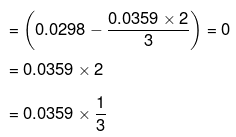Mole of FeO formed = 0.0359 × 2
Weight of FeO formed = 0.0359 × 2 × 72
= 5.17 g
The limiting reagent is one which is used completely, i.e. Fe2O3 here.

➢ Try Yourself!

Q.1. What is the number of moles of Fe(OH)3 (s) that can be produced by allowing 1 mole of Fe2S3, 2 moles of H2O and 3 moles of O2 to react?
2Fe2S3 (s) + 6H2O(l) + 3O2(g ) → 4Fe (OH )3(s) + 6S(s)
Ans. 1.34 moles

Q.2. In a process for producing acetic acid, oxygen gas is bubbled into acetaldehyde containing manganese (II) acetate (catalyst) under pressure at 60ºC.
2CH3CHO + O2 →2CH3COOH
In a laboratory test of this reaction, 20 g of CH3CHO and 10 g of O2 were put into a reaction vessel.
(a) How many grams of CH3COOH can be produced?
(b) How many grams of the excess reactant remain after the reaction is complete?
Ans.
(a) 27.27 g
(b) 2.73 g

➢ Concentration of Solution

The concentration terms are divided into two types.
1. Temperature dependant- the concentration terms that have a volume term, are affected by change in temperature. Example- Molarity, Normality etc
2. Temperature independant - the concentration terms that do not have a volume term and only mass/moles do not depend upon temperature. Example- Molality, mole fraction etc.

The concentration of a solution can be expressed in any of the following ways:
(a) % by Weight: Amount of solute dissolved in 100 gm of solution.
4.9% H2SO4 by weight: 100 gm of solution contains 4.9 gm of H2SO4.
(b) % by Volume: Volume of solute dissolved in 100 ml of solution.
x% H2SO4 by volume: 100 ml of solution contains x ml H2SO4
(c) % Weight by Volume: Weight of solute present in 100 ml of solution.
(d) % Volume by Weight: Volume of solute present in 100 gm of solution.

➢ Concentration Terms

• Molarity (M): No. of moles of solute present in 1000 ml of solution.
Molarity (M) = moles of solute/volume of solution (lit)
M = m.moles of solute/volume of solution (ml)
• Molality (m): No. of moles of solute present in 1000 gm of solvent.
m = moles of solute/wt. of solvent in kg
m = m.moles of solute/wt. of solvent in gm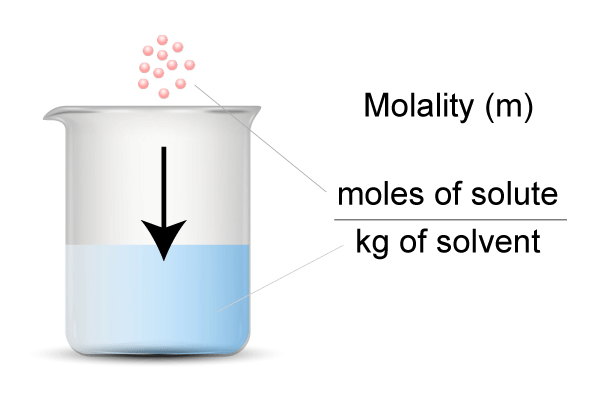• Normality (N): No of gm. equivalents of solute present in 1000 ml of solution.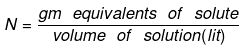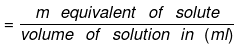Number of gram equivalent  of solute  = Mass of solute in gram/equivalent weight of solute
• Equivalent weight of solute (E) = Molar mass of solute / Valence factor
(i) Valence factor for base = acidity of base
(ii) Valence factor for acid = basicity of acid
(iii) Valence factor for element = valency
Thus, if one gram equivalent of a solute is present in one liter of the solution, the concentration of the solution is said to be one normal.

The relationship between Normality and Molarity can be expressed as:
► Molarity × Molecular mass = Strength of solution (g/L)
► Normality × Equivalent mass = Normality of the solution (g/L)
► Molarity × Molecular mass = Normality × Equivalent mass
Normality = n × Molarity

• Formality (f): It is the number of formula mass in grams present per litre of solution. In case formula mass is equal to molecular mass, formality is equal to molarity. Like molarity and normality, the formality is also dependent on temperature. It is used for ionic compounds in which there is no existence of a molecule. The mole of ionic compounds is called formole and molarity as formality.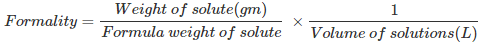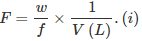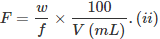Where,
w = weight of solute,
f = formula weight of solute
V = volume of solution
nf = no. of gram formula weight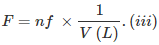• Mole Fraction: The mole fraction of a particular component in a solution is defined as the number of moles of that component per mole of solution. If a solution has nA mole of A & nB mole of B.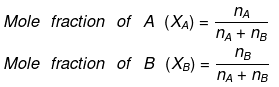And, XA + XB = 1
• Parts per million (ppm):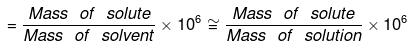• Volume strength of H2O2The strength of H2O2 is represented as 10V, 20V, 30V etc.
20V H2O2 means one litre of this sample of H2O2 on decomposition gives 20 lit. of O2 gas at S.T.P.
Decomposition of H2O2 is given as: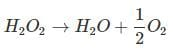1 mole = 1/2 × 22.4 lt O2 at S.T.P.
⇒ 34 g = 11.2 lt O2 at S.T.P.
To obtain 11.2 litre O2 at S.T.P., at least 34 gm H2O2 must be decomposed, and for 20 lt O2, we should decompose at least 34/112 × 20 gm H2O2.
1 lt solution of H2O2 contains 34/112 × 20  gm H2O2
1 lt solution of H2O2 contains 34/112 × 20/17 equivalents of H2O2
(E H2O2 = M/2 = 34/2 = 17)
Normality of H2O2 = 34/112 × 20/17
Normality of H2O(N)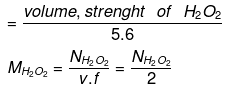Another Method: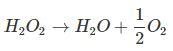From law of equivalence we have:
gm eq. of O2 = gm eq. of H2O2
gm eq. of O2 = moles × n factor of O2, = 20/22.4 x 4 = 20/5.6
gm. eq. of H2O2 = 20/5.6
and the volume of H2O2 is 1 lit.
this means 1 lit of H2O2 has 20/5.6 gm eq.
i.e. Normality N = 20/5.6
Normality of H2O2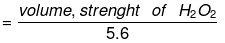Molarity of H2O(M)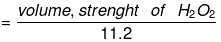Strength (in g/l): Denoted by S.
Strength = molarity × mol. wt.
= molarity × 34
strength = Normality × Eq. weight.
= Normality × 17

► Strength of Oleum
Oleum is SO3 dissolved in 100% H2SO4. Sometimes, oleum is reported as more than 100% by weight, say y% (where y > 100). This means that (y - 100) grams of water, when added to 100 g of given oleum sample, will combine with all the free SO3 in the oleum to give 100% sulphuric acid.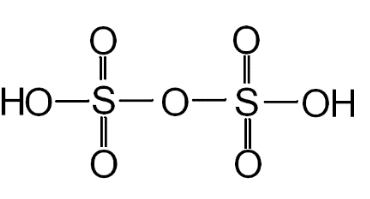Structure of Oleum

► Relationship between Molarity, Molality & Density of Solution
Let the molarity of solution be 'M', molality be 'm' and the density of solution be d gm/m.
Molarity implies that there are M moles of solute in 1000 ml of solution.
wt. of solution = density × volume
= 1000 d gm wt of solute = MM1
where M1 is the molecular wt of solute.
wt of solvent = (1000 d - MM1) gm.
(1000 d - MM1) gm of solvent contains M moles of solute.
1000 gm of solvent have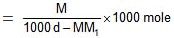=  Molality no. of moles of solute present in 1000 gm of solvent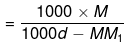= Molality on simplifying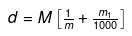► Relationship between Molality & Mole Fraction
Consider a binary solution consisting of two components A (Solute) and B (Solvent).
Let xA & xB are the mole fraction of A & B respectively.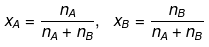If molality of solution be m then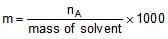=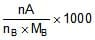where MB is the molecular wt of the solvent B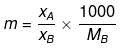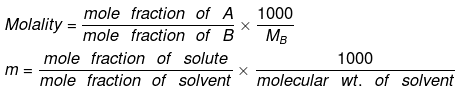Example 5. A bottle labeled with "12V H2O2" contains 700 ml solution. If a student mix 300 ml water in it what is the g/ liter strength & normality and volume strength of the final solution.
Solution. N = 12/5.6
Meq. of H2O2 = 12/5.6 x 100
let the normality of H2O2 on dilution be N.
Meq. before dilution = Meq. after dilution
N × 1000 = 12/5.6 x 100 N = 12/5.6 × 7/10 = 1.5 M = 1.5/2
strength gm/lit = 1.5/2 x 34 = 25.5
volume strength = N × 5.6 =  8.4 V

Example 6. Calculate the percentage of free SO3 in oleum (considered as a solution of SO3 in H2SO4) that is labeled '109% H2SO'.
Solution'109% H2SO4' refers to the total mass of pure H2SO4, i.e., 109 g that will be formed when 100 g of oleum is diluted by 9 g of H2O which (H2O) combines with all the free SO3 present in oleum to form H2SO4.
H2O + SO3 → H2SO4
1 mole of H2O combines with 1 mole of SO3
or 18 g of H2O combines with 80 g of SO3
or 9 g of H2O combines with 40 g of SO3.
Thus, 100 g of oleum contains 40 g of SO3 or oleum contains 40% of free SO3.

Example 7. A 62% by mass of an aqueous solution of an acid has a specific gravity of 1.8. This solution is diluted such that the specific gravity of the solution became 1.2. Find the % by wt of acid in the new solution.
SolutionDensity = 1.8
Volume of solution = Let x gm water be added in solution then
d = 0.6 x = 100
⇒ x = 166.67
Mass of new solution = 100 + 166.67 = 266.67
266.67 gm solution contains 62 gm of acid
% by mass = 23.24 %

Example 8. An aqueous solution is 1.33 molal in methanol. Determine the mole fraction of methanol & H2O.
Solution.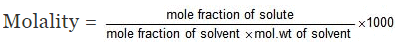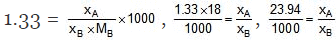⇒ xA = 0.02394 xB, xA xB = 1
⇒ 1.02394 xB = 1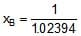= 0.98, xA = 0.02

Second Method: Let wt. of solvent = 1000 gm, molality = 1.33

= moles of solute
mole fraction of solute =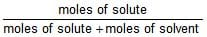,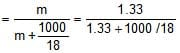mole fraction of solute = 0.02

mole fraction of solvent = 1 - 0.02 = 0.98

Example 9. The density of 3 M solution of sodium thiosulphate (Na2S2O3) is 1.25 g/mL. Calculate
(i) amount of sodium thiosulphate
(ii) mole fraction of sodium thiosulphate
(iii) molality of Na  and S2O32- ions
Solution.
(i) Let us consider one litre of sodium thiosulphate solution
wt. of the solution = density × volume (mL)
= 1.25 × 1000 = 1250 g.
wt. of Na2S2O3 present in 1 L of the solution
= molarity × mol. wt.
= 3 × 158 = 474 g.
wt. % of Na2S2O3 = 37.92%

(ii) Mass of 1 litre solution = 1.25 × 1000 g = 1250 g
[∵ density = 1.25 g/l]
Mole fraction of Na2S2O3 = Number of moles of Na2S2O3/Total number of moles
Moles of water = 1250 – 158 × 3/18 = 43.1
Mole fraction of Na2S2O3 = 3/3 + 43.1 = 0.065

(iii) 1 mole of sodium thiosulphate (Na2S2O3) yields 2 moles
of Na+ and 1 mole of S2O32-
Molality of Na2S2O3 = 3 × 1000/776 = 3.87
Molality of Na+ = 3.87 × 2 = 7.74 m
Molality of S2O2-3 = 3.87 m

Example 10. A solution of NaCl 0.5% by wt. If the density of the solution is 0.997 g/ml, calculate
(a) The molality
(b) Molarity
(c) Normality
(d) Mole fraction of the solute

Solution.
Number of moles of NaCl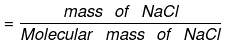= 0.5/58.5 = 0.00854

(a) By definition,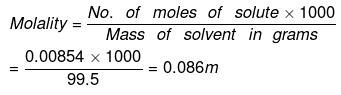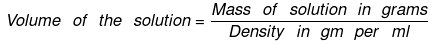= 100/0.997 = 100.3
(b) Now Molarity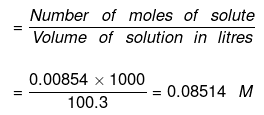(c) Normality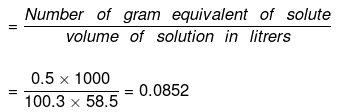(d) To calculate mole fraction of the solute
No. of moles of water in 99.5 g = 99.5/18 = 5.5277
Moles of NaCl = 0.5/58.5 = 8.547 x 10-3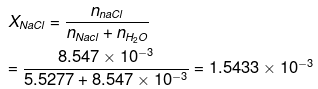XH2O = 1 - XNaCI = 0.9984

Example 11. Find the molarity and molality of a 15% solution of H2SO4 (density of H2SO= 1.020 g cm− 3) (Atomic mass: H = 1, O = 16, S = 32 amu).
Solution. 15% of a solution of H2SO4 means 15 g of H2SO4 are present in 100 g of the solution i.e.
Mass of H2SO4 dissolved = 15 g
Mass of the solution = 100 g
Density of the solution = 1.02 g/cm3 (given)
Calculation of molality:
Mass of solution = 100 g
Mass of H2SO4 = 15g
Mass of water (solvent) = 100 – 15 = 85 g
Mol. mass of H2SO4 = 98
∴ 15 g H2SO4 = 15/98 = 0.153 moles
Thus 85 g of the solvent contain 0.153 moles 100 g of the solvent containing
= 0.153/85 × 1000 = 1.8
Hence the molality of H2SO4 solution = 1.8 m
Calculation of molarity:
15 g of H2SO4 = 0.153 moles
Vol. of solution = W t. of solution/Density of solution = 100/1.02 = 98.04 cm3
Thus 98.04 cmof solution contain H2SO4 = 0.153 moles

Tip: Practice makes a man perfect. Practice as many questions as you can on this topic. Make handwritten short notes for the formulas!

The document Stoichiometry & Stoichiometric Calculations and Concentration Terms | Chemistry Class 11 - NEET is a part of the NEET Course Chemistry Class 11.
All you need of NEET at this link: NEET
FAQs on Stoichiometry & Stoichiometric Calculations and Concentration Terms | Chemistry Class 11 - NEET
 1. What is stoichiometry and why is it important?Ans. Stoichiometry is the branch of chemistry that deals with the quantitative relationships between reactants and products in chemical reactions. It is important because it allows us to predict how much product will be formed from a given amount of reactant and vice versa. This information is crucial for designing and optimizing chemical reactions in various fields such as pharmaceuticals, materials science, and environmental engineering.
 2. How do you calculate stoichiometric ratios?Ans. Stoichiometric ratios can be calculated by balancing the chemical equation for the reaction first, then determining the mole ratios between the reactants and products. For example, in the reaction 2H2 + O2 -> 2H2O, the stoichiometric ratio of hydrogen to oxygen is 2:1 because it takes two moles of hydrogen to react with one mole of oxygen to form two moles of water.
 3. What are some common concentration terms used in stoichiometry?Ans. Some common concentration terms used in stoichiometry include molarity, molality, percent by mass, and percent by volume. Molarity is defined as the number of moles of solute per liter of solution, while molality is defined as the number of moles of solute per kilogram of solvent. Percent by mass is the mass of the solute divided by the mass of the solution, multiplied by 100%, while percent by volume is the volume of the solute divided by the volume of the solution, multiplied by 100%.
 4. How do you perform stoichiometric calculations involving limiting reagents?Ans. Stoichiometric calculations involving limiting reagents require identifying which reactant is limiting and which is in excess. This can be done by comparing the mole ratios of the reactants to the actual mole amounts given in the problem. Once the limiting reagent is identified, the theoretical yield of the product can be calculated based on the mole ratio of the limiting reagent to the product. The actual yield can then be compared to the theoretical yield to calculate the percent yield.
 5. What is the difference between stoichiometry and stoichiometric calculations?Ans. Stoichiometry is the broad field of chemistry that deals with the quantitative relationships between reactants and products in chemical reactions, while stoichiometric calculations are specific calculations that use stoichiometry to determine the amounts of reactants and products in a given reaction. Stoichiometric calculations involve performing calculations using mole ratios, stoichiometric coefficients, and various concentration terms to calculate unknown quantities.

## Chemistry Class 11

199 videos|330 docs|229 tests

## Chemistry Class 11

199 videos|330 docs|229 tests

### How to Prepare for NEET

Read our guide to prepare for NEET which is created by Toppers & the best Teachers

Track your progress, build streaks, highlight & save important lessons and more!(Scan QR code)

,

,

,

,

,

,

,

,

,

,

,

,

,

,

,

,

,

,

,

,

,

;